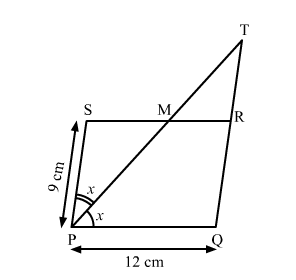# In a parallelogram PQRS, PQ = 12 cm and PS = 9 cm.

Question:

In a parallelogram PQRSPQ = 12 cm and PS = 9 cm. The bisector of ∠P meets SR in MPM and QR both when produced meet at T. Find the length of RT.

Solution:Given: In parallelogram PQRSPQ = 12 cm and PS = 9 cm. The bisector of ∠SPQ meets SR at M.

Let ∠SPQ = 2x.

⇒ ∠SRQ = 2x and ∠TPQ x.

Also, PQ ∥ SR

⇒ ∠TMR = ∠TPQ x.

In △TMR, SRQ is an exterior angle.

⇒ ∠SRQ = ∠TMR + ∠MTR

⇒ 2+ ∠MTR

⇒ ∠MTR x

⇒ △TPQ is an isosceles triangle.

⇒ TQ = PQ = 12 cm

Now,

RT TQ − QR

= TQ − PS

= 12 − 9

= 3 cm